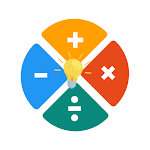ID: com.edustudionu.funbrainmathpuzzles

• Author:

• Version:

Varies with device

• Update on:

## The description of Fun Brain Math Puzzles

Various puzzles and types of Mathematics Problem Answers are solved here.
Let's fun for learn. This app provides you to do both math calculations and thinking logical. Challenge yourself with different levels of math puzzles.

Play these puzzles with your friends to see who can solve the puzzles faster.
Math puzzles increases your logical thinking. Beside that, you are be able to try the new biology quiz, chemistry, physics and zoology quiz to increase your knowledge.

The Fun Brain Math Puzzle game has some nice features:
1. More 100 Unique Levels math operations, you will find there: Addition puzzles, Subtraction, Multiplication, Division and more.
2. Brain games is based on principles of cognitive psychology to help you practice different mental skill: memory, attention, speed, reaction, concentration, logic and more.
3. You will find math solution step by step.

This app provides solution of all Math. This math puzzle will help you to learn math fun and easy. With explanation step by step, math solver, formulas, and mathematics trick,
make you fun and easy to learn mathematics.

Main features of this app
1. Very simple and easy to use,
2. Elegant design make you more interesting to learn maths.
2. Just few clicks to get a maths Solver.
3. Learn Mathematics by interesting Quiz.